Now that we’ve learned the representation of notes in sheet music, the time has come to study the note values. How can we know the time/duration of each note or chord in sheet music? By learning note values! See them below:

## The note values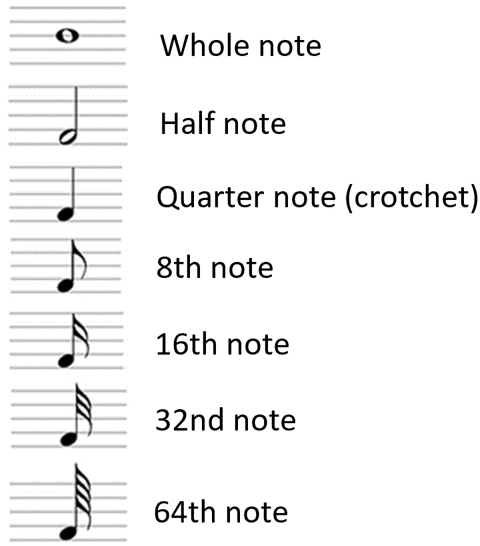Nice, but what is the time length of each one of these figures in practice? We’ll see that now. First of all, know that we have just shown the figures, in order, using the following logic: each figure shown lasts half the time of the previous figure. In other words, the Half note lasts half the time of the Whole note. The Quarter note, lasts half the time of the Half note and so on.

Let’s give you an example: if we assigned any value to these figures, let’s say, for example, that the value of the Quarter note is 1, we would have the following: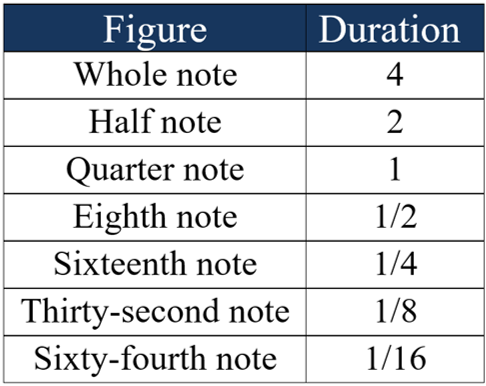We chose the value 1 for the quarter note here just to have a reference. Let’s make it more practical now by taking it to real life. Let’s say the value of a quarter note is US\$ 1.00. This would mean that:

• The Eighth Note is worth US\$ 0.50
• The Half Note is worth US\$ 2.00
• The Sixteenth Note is worth US\$ 0.25

etc.

This example was important to introduce the idea that a Quarter Note can fit 2 Eighth Notes or 4 Sixteenth Notes or 8 Thirty-second Notes, etc. Please note below: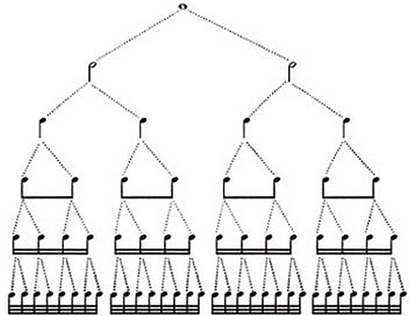Note: notice that the figures faster than the quarter note appear linked, forming blocks that correspond to one quarter note. For example, two eighth notes, one next to the other,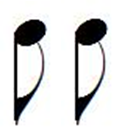are linked as follows: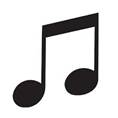In this diagram, it is easy to see how the figures fit inside each other. Note that 32 Thirty-second notes can fit within a Whole note. Therefore, if the 32th note represented 1 second, a Whole note would represent 32 seconds.

Great, this example served for teaching purposes, but how do we know the real time (in seconds) that each figure represents? How do you know if the Thirty-second note is worth 1 second, for example? Is there any definition in this regard?

Yes, there is. Obviously, we need a time reference for these figures to make sense. This reference will be provided by the song itself.

For example, let’s say you downloaded sheet music from the internet. Somewhere on the sheet music there will be how long a certain figure is worth (usually the value of a quarter note is informed) and the length of the other figures you will know by deduction.

This length is given in bpm (beats per minute), that is, if it is written:

this means that a Quarter note is worth 120 beats per minute.

To say “120 beats per minute” means to say that the length of each beat or note is such that 120 of these can fit within a minute. Since one minute has 60 seconds, this is the same as saying “2 beats per second”. Going further, we can conclude that a beat/note lasts half a second in this case.

Okay, but why don’t we say that a Quarter note is worth half a second instead of saying that it is worth “120 bpm”? This is because metronomes work in bpm. The best way to play a song from sheet music is having a metronome beside you where you can set the length of the beats.

In the example we just gave, you would set the metronome to 120 bpm and use this time (listening to the metronome) for each quarter note of the song on that sheet music. That is, while the metronome plays, the interval between one beat and another on the metronome represents the length of a quarter note (it is the length that the note should sound). If any notes appeared with the figure of an eighth note, you would leave that note sounding half the time of a quarter note.

## Tips to practice with a metronome

So it is important to train the following (with a metronome): Set a slow tempo, for example, 30 bpm (a beat lasts two seconds in this case). Start by playing one note at a time, that is, play your instrument, clap your hands, snap your fingers, tap your foot, whatever suits you, every time the metronome plays a beat.

Then play two notes at a time, that is, clap each time the metronome plays and also clap in the interval between one beat and another beat from the metronome. That way you will be doubling the speed of your claps. Then, do the same by playing 4 notes at a time (clap 4 times each time the metronome plays once, at equally spaced intervals). Repeat this exercise for other movements (40 bpm, 50 bpm, etc.) and practice the other figures as well. This is a great exercise.

With a little practice, you’ll be ready to get the right the length for a certain figure automatically when you read sheet music. We will give you other exercise tips below, but before that let’s learn a little more about the symbology in sheet music.

## How to link figures together

We’ve already seen that figures faster than quarter notes (such as eighth notes, sixteenth notes, etc.) appear connected in blocks when there are two or more of these figures in a sequence. However, different figures often appear in a sequence that is different among them, for example: 2 sixteenth notes and 1 eighth note. In this case, we know that one whole block (which is equivalent to a quarter note) would be formed by 4 sixteenth notes or 2 eighth notes. Observe:

4 sixteenth notes: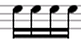2 eighth notes: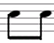But since 2 sixteenth notes are worth 1 eighth note, a block can also be formed by 2 sixteenth notes + 1 eighth note. And what would the representation of that block look like? Observe: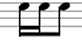Notice that the two sixteenth notes are connected to an eighth note forming a block of 3 notes that is equivalent to a quarter note.

Okay, now let’s make a mess in this block. Imagine that we are still with 2 sixteenth notes and 1 eighth note, but now the sixteenth notes are not next to each other. Let’s say the order is: sixteenth noteeighth notesixteenth note rather than sixteenth notesixteenth noteeighth note. In this case, the representation would look like this: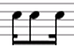Notice that the first note is connected to the second note with half the sixteenth note symbol and half the eighth note symbol: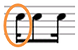This means that the first note must be played as a sixteenth note and the second as an eighth note. The idea is the same for the last note, which should be played as a sixteenth note: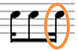Great, so this is how we represent the mixed blocks (with different figures). Now that we know the note values, the length of each one and the representations for different combinations of these notes, it is time to learn what a bar is.

Back to: Sheet music guide